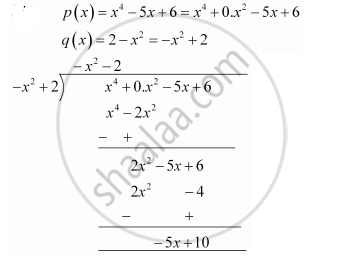# Divide the polynomial p(x) by the polynomial g(x) and find the quotient and remainder in each of the following - Mathematics

Divide the polynomial p(x) by the polynomial g(x) and find the quotient and remainder in each of the following

p(x) = x4 – 5x + 6, g(x) = 2 – x2

#### SolutionQuotient = - x2 - 2

Remainder = - 5x +10

Concept: Division Algorithm for Polynomials
Is there an error in this question or solution?
Chapter 2: Polynomials - Exercise 2.3 [Page 36]

#### APPEARS IN

NCERT Class 10 Maths
Chapter 2 Polynomials
Exercise 2.3 | Q 1.3 | Page 36

Share